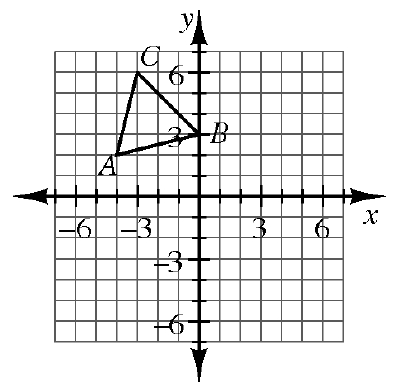### Home > INT2 > Chapter 1 > Lesson 1.2.4 > Problem1-62

1-62.Copy $ΔABC$ below on graph paper, then read the Math Notes box in this lesson before completing the parts below.

1. Rotate $ΔABC\ 90^\circ$ counterclockwise (↺) about the origin to create $ΔA'B'C'$. Name the coordinates of $C'$.

2. Reflect $ΔABC$ across the vertical line $x=1$ to create $ΔA''B''C''$. Name the coordinates of the vertices.

3. Translate $ΔABC$ so that $A'''$ is at $(4,\ -5)$. Name the coordinates of $B'''$.

Use the eTool below to view the rotations for parts (a), (b), and (c).
Click the link at right for the full version of the eTool: Int2 1-62 HW eTool